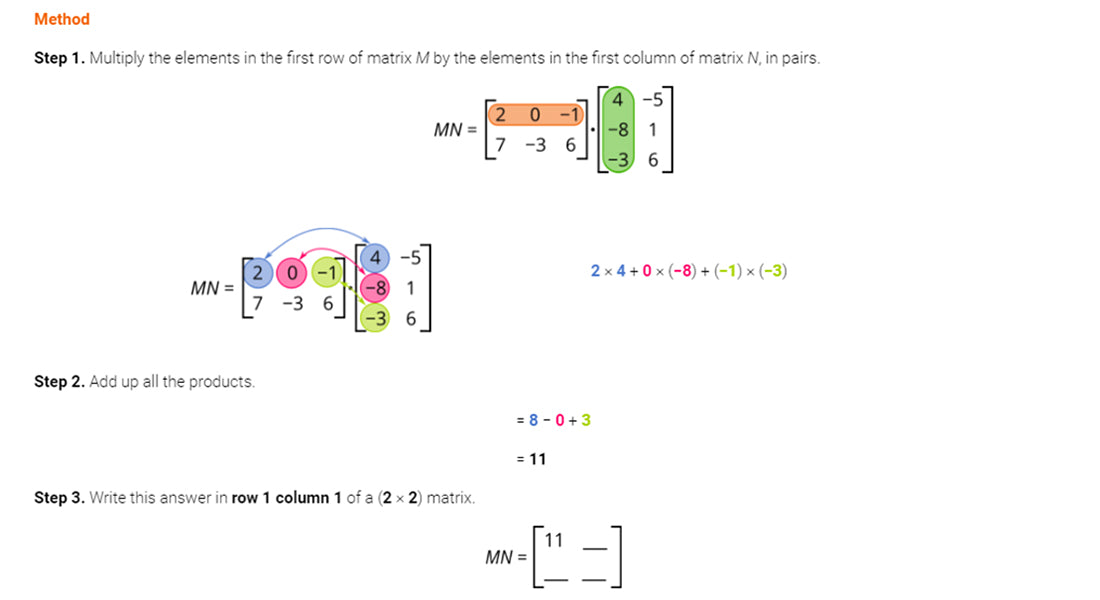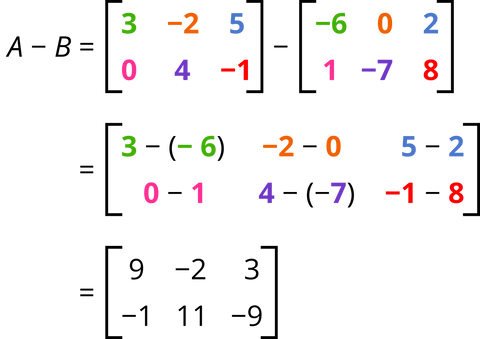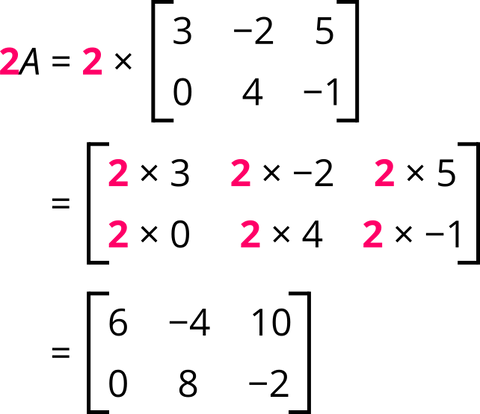# Further Mathematics - Exam Revision## Operations with Matrices

Authored By Carmen Popescu-Rose 0 Comment(s)

### Addition and subtraction of matrices

Requirement: Matrices must be of the same order.

How to: Corresponding elements are added (in addition) or subtracted (in subtraction).

Example

Consider the two matrices given below.a. Calculate the matrix AB.

The two matrices are of the same order, (2 × 3),  so they can be added.

b. Calculate the matrix A − B.

The two matrices are of the same order, (2 × 3),  so they can be subtracted.### Multiplying a matrix by a scalar

A scalar is any real number.

When multiplying a matrix by a scalar, each element of the matrix is multiplied by the scalar.

Example

Calculate the matrix 2A.### Multiplying matrices

Requirement: Matrices can be multiplied if the number of columns in the first matrix is equal to the number of rows in the second matrix.

Example

The order of matrix M is (a × b), where a and b are positive integers.

The order of matrix N is (b × c), where b and c are positive integers.

The product MN is defined because the number of columns in matrix M is equal to the number of rows in the matrix N.

(a × b)(b × c)

The order of the product matrix is (a × c).

The product MN is not always equal to the product NM.

How to: This can be easily explained using an example.

Example

Consider the two matrices given below.andThe order of the matrix M is (2 × 3), where a and b are positive integers.

The order of the matrix N is (3 × 2), where b and c are positive integers.

The product MN is defined because the number of columns in matrix M is equal to the number of rows in the matrix N.

(2 × 3) (3 × 2)

The order of the product matrix is (2 × 2).

Method

Step 1. Multiply the elements in the first row of matrix M by the elements in the first column of matrix N, in pairs.2 × 4 + 0 × (−8) + (−1) × (−3)

Step 2. Add up all the products.

= 80 + 3

= 11

Step 3. Write this answer in row 1 column 1 of a (2 × 2) matrix.Step 4. Repeat Steps 1 − 3 for the multiplication of row 1 and column 2.2 × (−5) + 0 × 1 + (−1) × 6 = −10 + 0 + (−6)                                               = − 16

Step 5. Write this answer in row 1 column 2 in the (2 × 2) matrix.Step 6. Repeat Steps 1 − 3 for the multiplication of row 2 and column 1.7 × 4 + (−3) × (−8) + 6 × (−3) = 28 + 24 + (−18)                                                    = 34

Step 7. Write this answer in row 2 column 1 in the (2 × 2) matrix.Step 8. Repeat Steps 1 − 3 for the multiplication of row 2 and column 2.7 × (−5) + (−3) × 1 + 6 × 6 = (−35) + (−3) + 36                                                = −2

Step 9. Write this answer in row 2 column 2 in the (2 × 2) matrix.